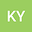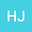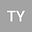Slope- watershed coupling simulation under different vegetation coverage based on GAST model
•••• +2Hou Jingming
Xi'an University of Technology
Author ProfileTong Yu
Xi'an University of Technology
Author ProfileShi Baoshan
Xi'an University of Technology
Author ProfilePan Zhanpeng
Xi'an University of Technology
Author Profile## Abstract

A non-equilibrium sediment transport soil erosion model based on finite volume method (FVM) coupled with two-dimensional hydrodynamic process is proposed, application of the GPU techniques in the numerical model, making it possible to simulate the sediment transport and bed evolution in a high resolution but efficient way. The first-order Gudonov format FVM is used to discreting the control equation. The variables on both sides of the unit interface are obtained by limiting slope interpolation. An efficient and robust non-negative depth reconstruction algorithm is used to solve the dry-wet boundary problem. This algorithm makes the model have second-order accuracy in space, and also effectively suppresses the numerical oscillation. Harten, Lax van Leer Contact (HLLC) approximate Riemann solver is used to calculate mass and momentum flux, and the friction source term is calculated by the proposed split point implicit method. These values are evaluated by a novel 2D edge-based MUSCL scheme. The code is programmed using C++ and CUDA, which can be run on GPU to greatly accelerate the calculation speed. In this paper, two numerical experiments show that the model performs well in accuracy and robustness of the algorithm in the process of slope erosion and watershed erosion. The constructed model can simulate the soil erosion of slope and watershed gully under different vegetation coverage, and characterize the erosion process of interaction between slope and gully.